# Introduction to Data Visualization using Python

26 September 2017 — Written by Mubaris NK

Data visualization is one of primary skills of any data scientist. It's also a large field in itself. There are many courses available just focused on Data Visualization. This post is just an introduction to this much broader topic.

In this post first we will look at data visualization conceptually, then we will explore more using Python libraries.

## What is Data Visualization?

By visualizing information, we turn it into a landscape that you can explore with your eyes, a sort of information map. And when you’re lost in information, an information map is kind of useful. ―David McCandless

Data visualizations is the process of turning large and small datasets into visuals that are easier for the human brain to understand and process.

When we have a dataset, it will take some time to make the meaning of that data. But, when we represent this data in graphs or other visualizations, it is much more easier for us to understand. That's the power of data visualization.

### Examples of Data Visualizations

• Countries with largest defense budgetYou can clearly see that US defense budget almost equal as the combined budget of other countries.

• Largest Occupations in the United States• Atheists in Europe• Death by Heart Decease in US by Nick UsoffI can show you many more here. There are endless supply of Data Visualizations available on internet.

### Principles of Good Data Visualization

These principles are directly taken from Data Visualisation: A Handbook for Data Driven Design by Andy Kirk. I highly recommend reading this book.

• Trustworthy

This means that the data presented is honestly portrayed, or the visualization is not misleading. Trust is hard to earn and easy to lose. This is very important.

• Accessible

• Elegant

It's important to have stylish and beautiful visualization when you present them. If you are exploring data, it might not be critical. But, if you presenting your visualization to a particular audience or submitting on some platform, you will need beautiful visualizations.

## Data Visualization in Python using Matplotlib

Matplotlib is a widely used visualization package in Python. It's very easy to create and present data visualizations using Matplotlib. There are other visualization libraries available in Python.

We are going to learn how to create Bar plots, Line plots and Histograms using Matplotlib in this post. The entire code created is using Jupyter Notebooks.

### Line Plots

Line plots are very simple plots. It represents frequency of data along a number lines. You can learn more about Line charts and Spline charts from Data Viz Project.

We'll use Bitcoin Historical Price Dataset from Kaggle to draw line plots here.

First we'll import all numpy, pandas and matplotlib. Then we read the data using read_csv function from pandas.

import numpy as np
import pandas as pd
import matplotlib.pyplot as plt

data.head()

You will get an output table with 24 columns and 5 rows(Too long to print here).

data.shape
(1590, 24)

We'll need to convert the Date string to pandas datetime.

data['Date'] = pd.to_datetime(data['Date'].values)

Now we extract date and price from our data set.

date = data['Date'].values
price = data['btc_market_price'].values

Now we can plot using these values.

plt.plot(date, price)
plt.show()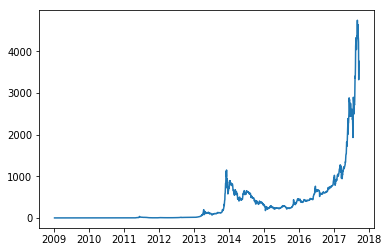This plot is not labelled. And the axes are not perfect. We'll fix that now.

plt.plot(date, price, c='magenta')

plt.title("BTC Price over time")

# Axis labels
plt.xlabel("Year")
plt.ylabel("Price in USD")

# Axes Range
plt.axis(['2009', '2018', 0, 5000])

plt.show()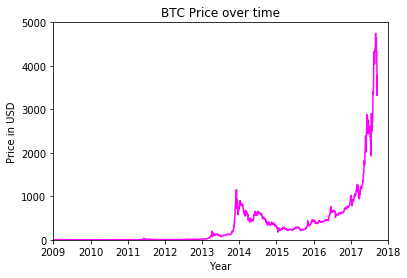### Bar Plots

Bar Plot is chart that represents categorical data with rectangular bars. More about bar plots at Data Viz Project

We'll use European Developers Salary data to plot bar graph. Get this data from here

At first we read the data from csv file.

salary = pd.read_csv('salary.csv')
salary.columns = ['Experience', 'Salary', 'Country']
salary.head()
Experience Salary Country
0 5.0 27930 Austria
1 21.0 28000 Austria
2 5.0 39200 Austria
3 0.0 39200 Austria
4 9.0 40000 Austria

We will be plotting mean salary by each country. So we'll get mean value by each country.

salary = salary.groupby(['Country']).mean()
salary
Experience Salary
Country
Austria 7.980000 53385.200000
Belgium 6.952381 55803.047619
Bulgaria 10.264706 42017.647059
Croatia 6.600000 30275.900000
Cyprus 3.000000 26093.333333
Czech Republic 8.562500 46110.750000
Denmark 9.562500 83223.666667
Estonia 7.153846 37526.153846
Finland 6.117647 45642.647059
France 5.507843 49085.176471
Germany 6.607735 66540.110497
Greece 8.769231 31716.153846
Hungary 7.722222 26873.666667
Ireland 7.414894 62754.510638
Italy 7.526923 34007.692308
Latvia 5.333333 32666.666667
Lithuania 7.200000 34333.333333
Luxembourg 12.750000 61250.000000
Malta 7.400000 48400.000000
Netherlands 6.890000 54096.537500
Norway 8.342105 107457.421053
Poland 6.322222 36655.111111
Portugal 5.300000 30148.500000
Romania 6.183333 35043.133333
Serbia 7.375000 33450.000000
Slovakia 4.400000 24618.000000
Slovenia 8.000000 37380.000000
Spain 7.070755 38556.452830
Sweden 6.792453 77481.000000
Switzerland 7.250000 93962.250000
United Kingdom 6.080214 68270.550802

Now we extract these values to plot. We are only taking first 5 countries.

country = salary.index[:5]
country_array = np.arange(5)
mean_salary = salary['Salary'].values[:5]
# Basic Plot
plt.bar(country_array, mean_salary, color='#f44c44')

# X-Axis Tick Labels
plt.xticks(country_array, country)

# Title
plt.title("European Developers Salary")

# Y-Axis Label
plt.ylabel("Salary in €")

plt.show()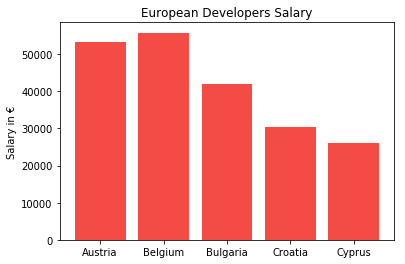We can clearly see that Belgium has the highest average salary and Cyprus has least average salary among these five countries.

### Histogram

Histogram a diagram consisting of rectangles whose area is proportional to the frequency of a variable and whose width is equal to the class interval. More about Histograms

We are going to generate some random numbers using numpy. Then we will plot histogram of these random numbers.

data = np.random.randint(low=-100, high=250, size=400)

#Plot. bins=no. of bins
plt.hist(data, bins=15, color='#988659')
plt.xlabel('Value')
plt.ylabel('Frequency')
plt.title('Histogram')

#Using Grids
plt.grid()

plt.show()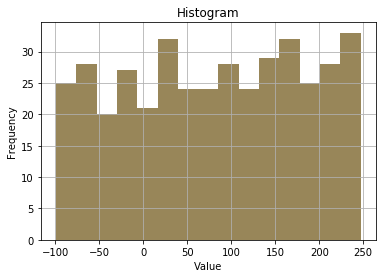This is not showing any kind of special data. We can generate Gaussian(Normal) random numbers using numpy to create better histograms. These are just random numbers, this doesn't represent any data.

# Mean = 5, Standard Deviation = 2, Number of points = 1000
data = np.random.normal(5, 2, 1000)

#Plot. bins=no. of bins
plt.hist(data, bins=10, color='#8cdcb4')
plt.xlabel('Value')
plt.ylabel('Frequency')
plt.title('Histogram')

#Using Grids
plt.grid()

plt.show()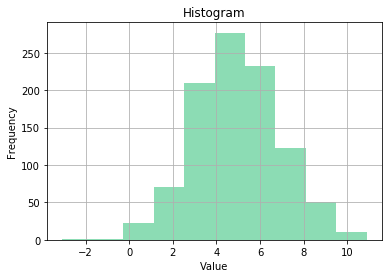## Conclusion

So far we have learned how to create Line plots, Bar plots and Histograms using Matplotlib library. In the future posts we will learn more about how to create more plots. Also, we will use data science methods for a particular case study.